## Python Tutorial

Python HOME Python Intro Python Get Started Python Syntax Python Comments Python Variables Python Data Types Python Numbers Python Casting Python Strings Python Booleans Python Operators Python Lists Python Tuples Python Sets Python Dictionaries Python If...Else Python While Loops Python For Loops Python Functions Python Lambda Python Arrays Python Classes/Objects Python Inheritance Python Iterators Python Scope Python Modules Python Dates Python Math Python JSON Python RegEx Python PIP Python Try...Except Python User Input Python String Formatting

## File Handling

Python File Handling Python Read Files Python Write/Create Files Python Delete Files

## Python Modules

NumPy Tutorial Pandas Tutorial SciPy Tutorial

## Python Matplotlib

Matplotlib Intro Matplotlib Get Started Matplotlib Pyplot Matplotlib Plotting Matplotlib Markers Matplotlib Line Matplotlib Labels Matplotlib Grid Matplotlib Subplots Matplotlib Scatter Matplotlib Bars Matplotlib Histograms Matplotlib Pie Charts

## Machine Learning

Getting Started Mean Median Mode Standard Deviation Percentile Data Distribution Normal Data Distribution Scatter Plot Linear Regression Polynomial Regression Multiple Regression Scale Train/Test Decision Tree

## Python MySQL

MySQL Get Started MySQL Create Database MySQL Create Table MySQL Insert MySQL Select MySQL Where MySQL Order By MySQL Delete MySQL Drop Table MySQL Update MySQL Limit MySQL Join

## Python MongoDB

MongoDB Get Started MongoDB Create Database MongoDB Create Collection MongoDB Insert MongoDB Find MongoDB Query MongoDB Sort MongoDB Delete MongoDB Drop Collection MongoDB Update MongoDB Limit

## Python Reference

Python Overview Python Built-in Functions Python String Methods Python List Methods Python Dictionary Methods Python Tuple Methods Python Set Methods Python File Methods Python Keywords Python Exceptions Python Glossary

## Module Reference

Random Module Requests Module Statistics Module Math Module cMath Module

## Python How To

Remove List Duplicates Reverse a String Add Two Numbers

## Python Examples

Python Examples Python Compiler Python Exercises Python Quiz Python Certificate

# Matplotlib Pie Charts

## Creating Pie Charts

With Pyplot, you can use the `pie()` function to draw pie charts:

### Example

A simple pie chart:

import matplotlib.pyplot as plt
import numpy as np

y = np.array([35, 25, 25, 15])

plt.pie(y)
plt.show()

### Result: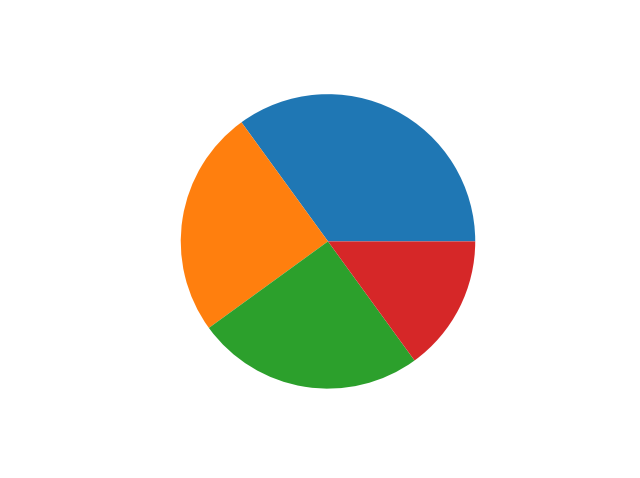Try it Yourself »

As you can see the pie chart draws one piece (called a wedge) for each value in the array (in this case [35, 25, 25, 15]).

By default the plotting of the first wedge starts from the x-axis and move counterclockwise: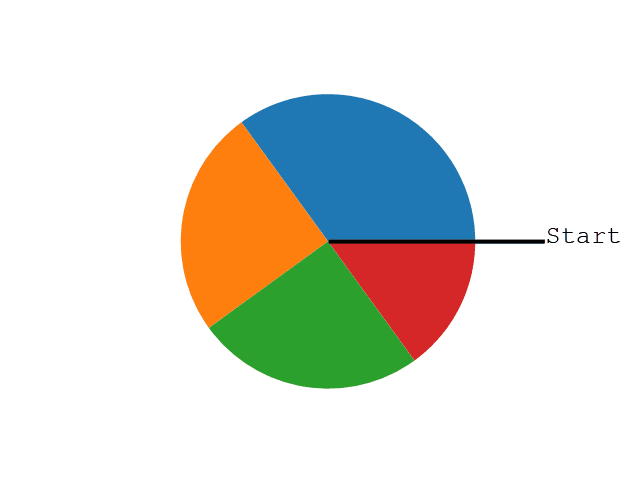Note: The size of each wedge is determined by comparing the value with all the other values, by using this formula:

The value divided by the sum of all values: `x/sum(x)`

## Labels

Add labels to the pie chart with the `label` parameter.

The `label` parameter must be an array with one label for each wedge:

### Example

A simple pie chart:

import matplotlib.pyplot as plt
import numpy as np

y = np.array([35, 25, 25, 15])
mylabels = ["Apples", "Bananas", "Cherries", "Dates"]

plt.pie(y, labels = mylabels)
plt.show()

### Result:Try it Yourself »

## Start Angle

As mentioned the default start angle is at the x-axis, but you can change the start angle by specifying a `startangle` parameter.

The `startangle` parameter is defined with an angle in degrees, default angle is 0: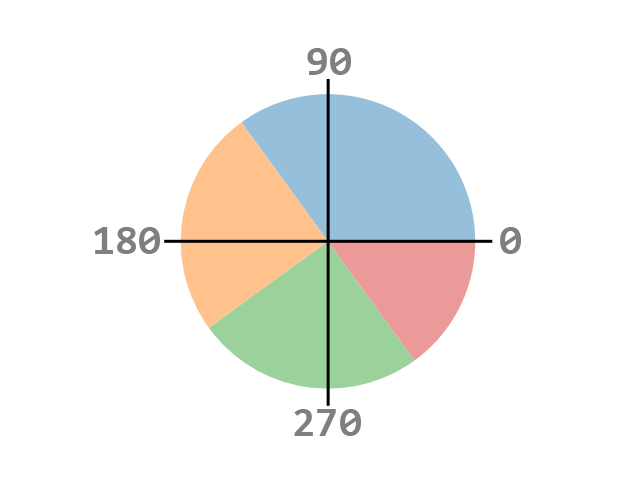### Example

Start the first wedge at 90 degrees:

import matplotlib.pyplot as plt
import numpy as np

y = np.array([35, 25, 25, 15])
mylabels = ["Apples", "Bananas", "Cherries", "Dates"]

plt.pie(y, labels = mylabels, startangle = 90)
plt.show()

### Result:Try it Yourself »

## Explode

Maybe you want one of the wedges to stand out? The `explode` parameter allows you to do that.

The `explode` parameter, if specified, and not `None`, must be an array with one value for each wedge.

Each value represents how far from the center each wedge is displayed:

### Example

Pull the "Apples" wedge 0.2 from the center of the pie:

import matplotlib.pyplot as plt
import numpy as np

y = np.array([35, 25, 25, 15])
mylabels = ["Apples", "Bananas", "Cherries", "Dates"]
myexplode = [0.2, 0, 0, 0]

plt.pie(y, labels = mylabels, explode = myexplode)
plt.show()

### Result: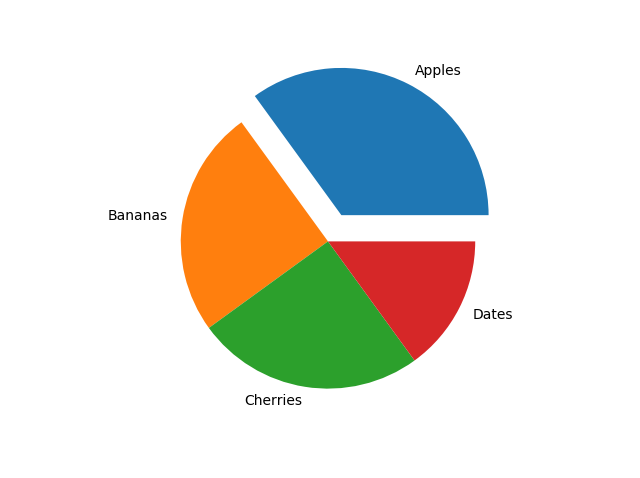Try it Yourself »

Add a shadow to the pie chart by setting the `shadows` parameter to `True`:

### Example

import matplotlib.pyplot as plt
import numpy as np

y = np.array([35, 25, 25, 15])
mylabels = ["Apples", "Bananas", "Cherries", "Dates"]
myexplode = [0.2, 0, 0, 0]

plt.pie(y, labels = mylabels, explode = myexplode, shadow = True)
plt.show()

### Result: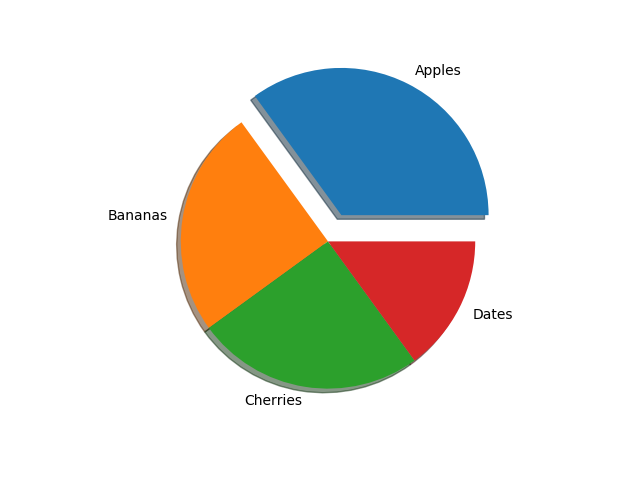Try it Yourself »

## Colors

You can set the color of each wedge with the `colors` parameter.

The `colors` parameter, if specified, must be an array with one value for each wedge:

### Example

Specify a new color for each wedge:

import matplotlib.pyplot as plt
import numpy as np

y = np.array([35, 25, 25, 15])
mylabels = ["Apples", "Bananas", "Cherries", "Dates"]
mycolors = ["black", "hotpink", "b", "#4CAF50"]

plt.pie(y, labels = mylabels, colors = mycolors)
plt.show()

### Result:Try it Yourself »

You can use Hexadecimal color values, any of the 140 supported color names, or one of these shortcuts:

`'r'` - Red
`'g'` - Green
`'b'` - Blue
`'c'` - Cyan
`'m'` - Magenta
`'y'` - Yellow
`'k'` - Black
`'w'` - White

## Legend

To add a list of explanation for each wedge, use the `legend()` function:

### Example

import matplotlib.pyplot as plt
import numpy as np

y = np.array([35, 25, 25, 15])
mylabels = ["Apples", "Bananas", "Cherries", "Dates"]

plt.pie(y, labels = mylabels)
plt.legend()
plt.show()

### Result: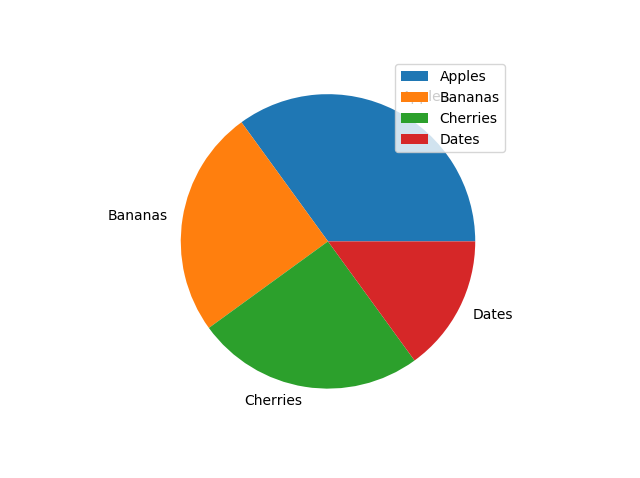Try it Yourself »

To add a header to the legend, add the `title` parameter to the `legend` function.

### Example

import matplotlib.pyplot as plt
import numpy as np

y = np.array([35, 25, 25, 15])
mylabels = ["Apples", "Bananas", "Cherries", "Dates"]

plt.pie(y, labels = mylabels)
plt.legend(title = "Four Fruits:")
plt.show()

### Result:Try it Yourself »